# Find the factor

1
One factor is 0
2
One factor is 1
3
One factor is 2
4
One factor is 3
5
One factor is 1-3
6
One factor is 4
7
One factor is 5
8
One factor is 6
9
One factor is 4-6
10
One factor is 7
11
One factor is 8
12
One factor is 9
13
One factor is 10
14
One factor is 7-10
15
One factor is 2-9
16
One factor is 1-10
17
One factor is 11
18
One factor is 12
19
One factor is 13
20
One factor is 11-13
21
One factor is 14
22
One factor is 15
23
One factor is 16
24
One factor is 14-16
25
One factor is 17
Extremely difficult
26
One factor is 18
27
One factor is 19
28
One factor is 20
29
One factor is 17-20
30
One factor is 11-20
Extremely difficult
31
One factor is 21-25
Extremely difficult
32
One factor is 26-30
Extremely difficult
33
One factor is 31-35
Extremely difficult
34
One factor is 36-40
Extremely difficult
35
One factor is 41-45
Extremely difficult
36
One factor is 46-50
Extremely difficult
37
One factor is 51-55
Extremely difficult
38
One factor is 56-60
Extremely difficult
39
One factor is 61-65
Extremely difficult
40
One factor is 66-70
Extremely difficult
41
One factor is 71-75
Extremely difficult
42
One factor is 76-80
Extremely difficult
43
One factor is 81-85
Extremely difficult
44
One factor is 86-90
Extremely difficult
45
One factor is 91-100
Extremely difficult
Do you want to play locked game level?

## Recent activities in this game

Someone got all questions correct at level 1. One factor is 0 .
20 hours ago after 6 seconds of playing.
Someone got all questions correct at level 1. One factor is 0 .
2023-12-08 21:11 after 6 seconds of playing.
Someone got all questions correct at level 1. One factor is 0 .
2023-12-07 18:22 after 6 seconds of playing.
Someone got all questions correct at level 1. One factor is 0 .
2023-12-06 22:06 after 7 seconds of playing.
Someone got all questions correct at level 1. One factor is 0 .
2023-12-05 21:14 after 6 seconds of playing.
Someone had a problem at level 2. One factor is 1 .
2023-12-05 20:16 after 2 minutes of playing.
Someone got all questions correct at level 1. One factor is 0 .
2023-12-05 20:14 after 49 seconds of playing.
Someone got all questions correct at level 1. One factor is 0 .
2023-12-05 02:53 after 7 seconds of playing.
Someone got all questions correct at level 1. One factor is 0 .
2023-12-05 00:51 after 7 seconds of playing.
Someone got all questions correct at level 1. One factor is 0 .
2023-12-03 22:56 after 6 seconds of playing.

Can you find the missing factorn in these multiplication problems?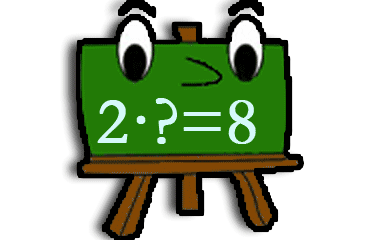## Game instructions to finding the factor

Choose a number from the buttons above. This will give you up to ten tasks where you get one number (factor 1) which multiplied with another unknown number (factor 2) results in the given product. If you can solve all the tasks, you will get a knowledge point and the number's button will be marked green.

We've got another game about multiplication where you are supposed to find the product. That game is usually a litte bit easier to start with. Or why not alternate levels from that game with levels in this game matched by the numbers?

Two factors multiplied with each other results in a product:
 3 · 2 = 6 factor · factor = product

### Calculation of knowledge points

Each completed game level gives 1 knowledge point in Find the factor. The maximum number of points (45 knowledge points) is achieved when you pass all 45 levels. You'll get a bronze medal when you complete a level 2 times and a silver medal after 5 completed rounds. A gold medal will be received after 10 completed rounds. In Find the factor, the maximum number of collectable gold medals is 45. You only earn knowledge points for levels that has not been cleared before. A cleared level has a green background above. Even when you have completed a level, you can continue practicing at that level, but it gives you no more knowledge points.

### Similar games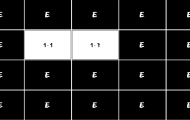Math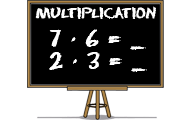Math

Subject
Math
Target group
Practices
Factor Multiplication Product
Game creator
Updated
2014-05-30
Published online
2014-05-30
Unique id
21
Saved by
0-5 users
Operating system
Browser
Price
Free of charge

3.9
Total 112
5
68
4
9
3
7
2
10
1
18

### Report

If you find a spelling mistake or something else that should be corrected, feel free to contact the game creator or us at Helpful Games.

Write a comment below to contact the game creator or

There is 1 comment on this knowledge game.

A
17 October 2020 19:11
Hi. This is a lovely multiplication games. Especially, I know what is 12 x 20 = 240!
But i would still say: I don't know the multiplication factors of 13. Some like 13 x 1 - 31 x 10 i know but i don't know what is 13 x 11 - 31 x 20. I know tables of 2, 5, 10, 6, 7, 8 , 9, 10, 11, 12, 15, 20, 21, 22, 25 and may other multiplication factors
Most played
1.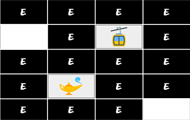Brain training
2.Brain training
3.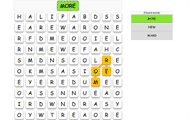English
4.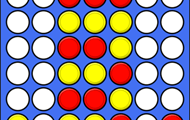Brain training
5.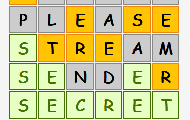English

More plus membership benefits